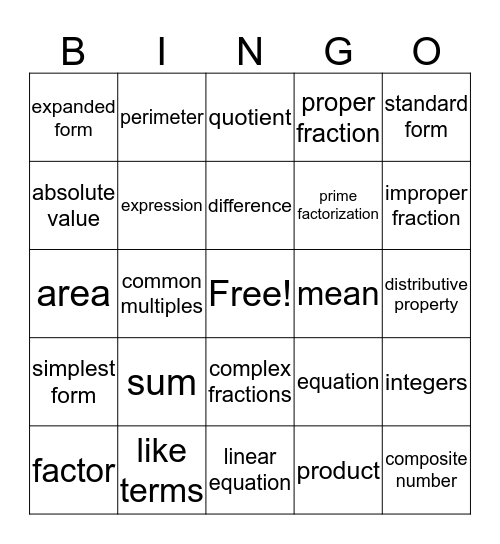# vocabulary BingoThis bingo card has a free space and 24 words: factor, sum, difference, product, standard form, expanded form, perimeter, quotient, mean, distributive property, area, expression, equation, integers, absolute value, like terms, linear equation, proper fraction, improper fraction, simplest form, common multiples, complex fractions, prime factorization and composite number.

## Play Online